# OECD Business confidence Index(BCI) data analysis 5 - Time-Series Regression using R, Finite Distributed Lag(FDL) ModelPhoto by JuniperPhoton on Unsplash

This post is follwong of abovr post.
In this post, I will examone Finite Distributed Lag(FDL) Model.

Firstly, I make a ts object from df_avg objrect.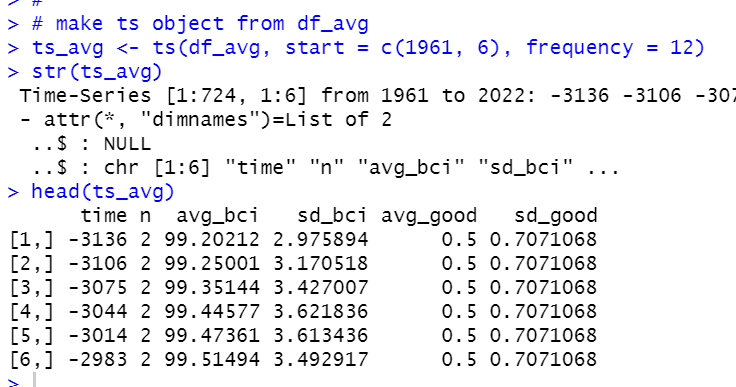Then, I use dynlm() function to make a FDL model.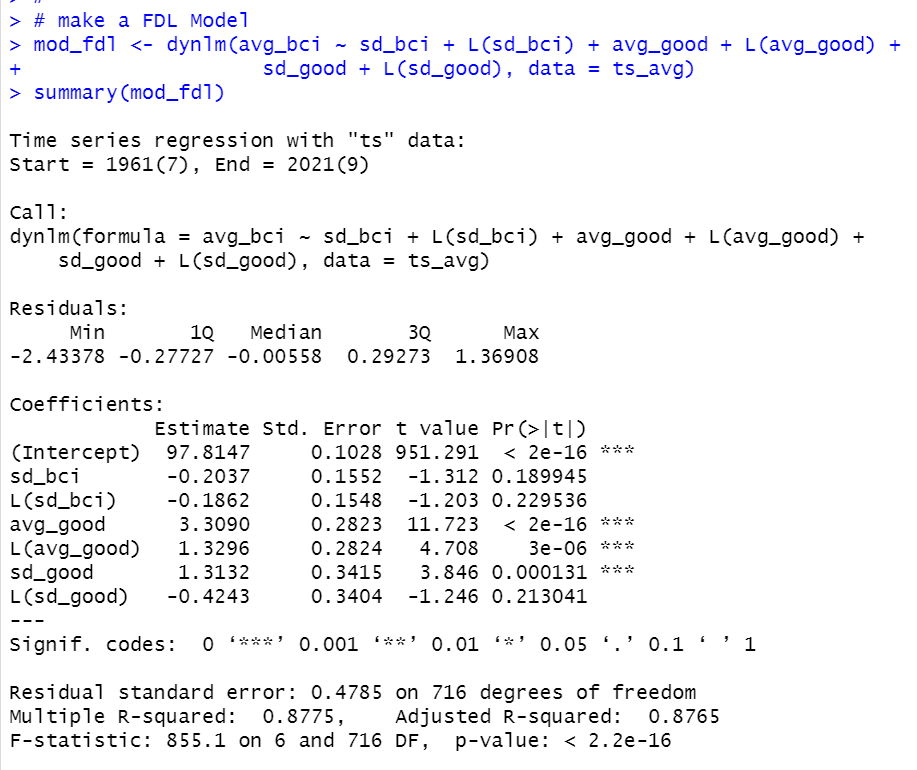Next, let's check if there is serial correlation.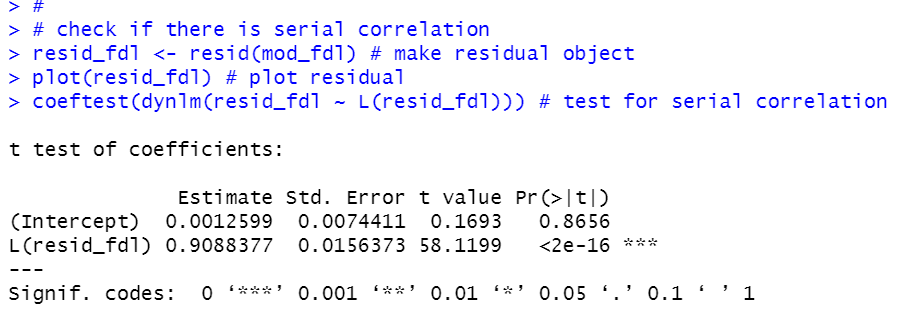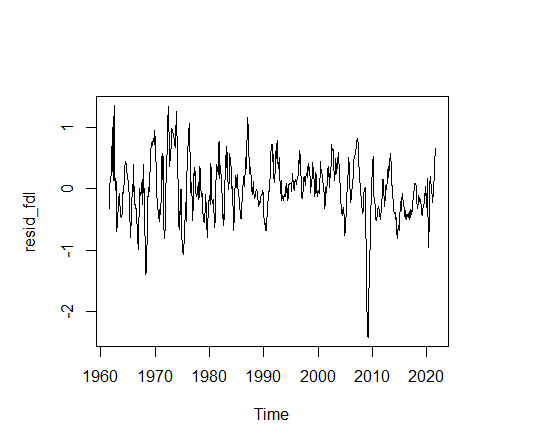L(resid_fdl) coefficient p-value is almost 0. We see there is serial correlation.

So, let's use Cochrane-Orcutt estimation using cochrane.orcutt() function.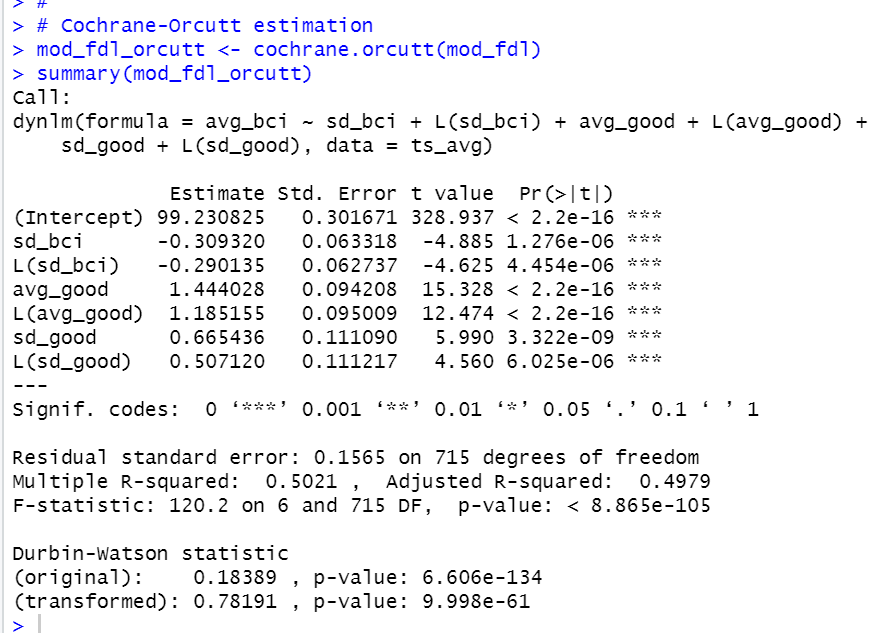All right, let's compare mod_fdl and mod_fdl_orcutt with stargazer() function.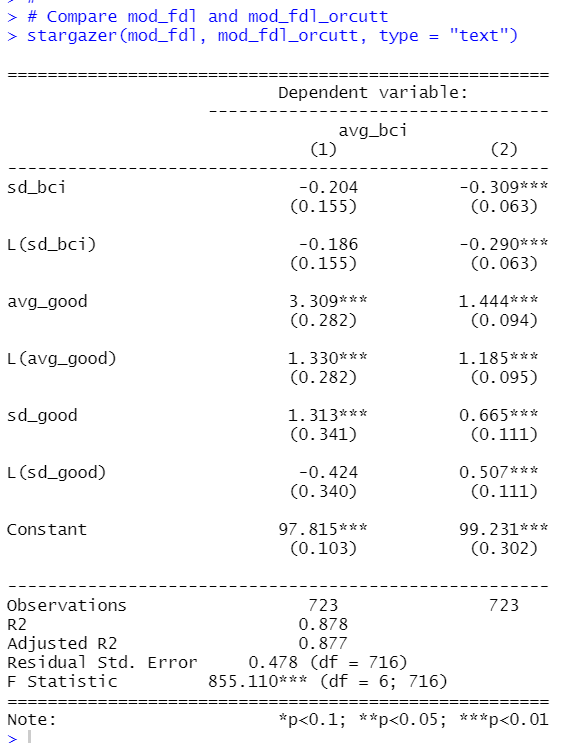mod_static, mod_orcutt, mod_fdl and mod_fdl_orcutt.

Let's compare those models' fitted values.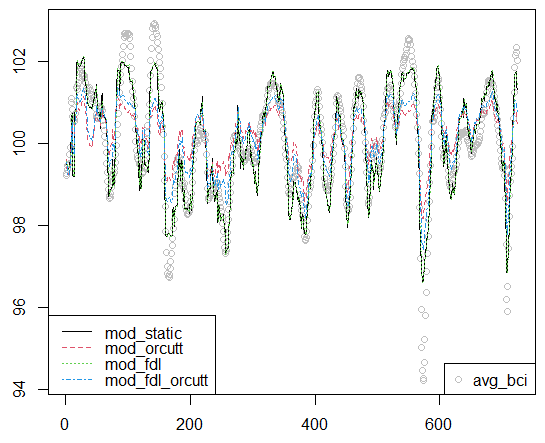All four models have good fitting, I think.
That's it. Thank you!

Next post is

To read from the 1st post,